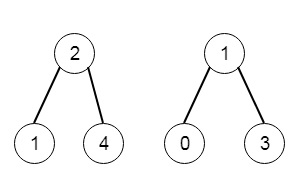# Two Sum BSTs in C++

Suppose we have two binary search trees, we have to return True iff there is a node in the first tree and a node in the second tree and sum of these nodes is a given integer target. So if the tree is like −and target is 5, then the result is true.

To solve this, we will follow these steps −

• Define a map s
• define a method called check(), this will take node, target and nodeNumber, this will work as follows −
• if node is valid, then return false
• curr := value of node, req := target – curr
• if req is present in s and s[req] is not nodeNumber, then return true
• s[curr] := nodeNumber
• return check(left of node, target, nodeNumber) OR check(right of node, target, nodeNumber)
• In the main method, we will set −
• flag := check(root1, target, 1)
• return check(root2, target, 2)

## Example(C++)

Let us see the following implementation to get a better understanding −

Live Demo

#include <bits/stdc++.h>
using namespace std;
class TreeNode{
public:
int val;
TreeNode *left, *right;
TreeNode(int data){
val = data;
left = NULL;
right = NULL;
}
};
void insert(TreeNode **root, int val){
queue<TreeNode*> q;
q.push(*root);
while(q.size()){
TreeNode *temp = q.front();
q.pop();
if(!temp->left){
if(val != NULL)
temp->left = new TreeNode(val);
else
temp->left = new TreeNode(0);
return;
}
else{
q.push(temp->left);
}
if(!temp->right){
if(val != NULL)
temp->right = new TreeNode(val);
else
temp->right = new TreeNode(0);
return;
}
else{
q.push(temp->right);
}
}
}
TreeNode *make_tree(vector<int> v){
TreeNode *root = new TreeNode(v);
for(int i = 1; i<v.size(); i++){
insert(&root, v[i]);
}
return root;
}
class Solution {
public:
map <int,int> s;
bool check(TreeNode* node, int target,int nodeNumber){
if(!node)return false;
int curr = node->val;
int req = target - curr;
if(s.find(req)!=s.end() && s[req]!=nodeNumber)return true;
s[curr]=nodeNumber;
return check(node->left,target,nodeNumber) || check(node->right,target,nodeNumber);
}
bool twoSumBSTs(TreeNode* root1, TreeNode* root2, int target) {
bool flag = check(root1,target,1);
return check(root2,target,2);
}
};
main(){
vector<int> v1 = {2,1,4};
vector<int> v2 = {1,0,3};
TreeNode *r1 = make_tree(v1);
TreeNode *r2 = make_tree(v2);
Solution ob;
cout <<ob.twoSumBSTs(r1, r2, 5);
}

## Input

[2,1,4]
[1,0,3]
5

## Output

1